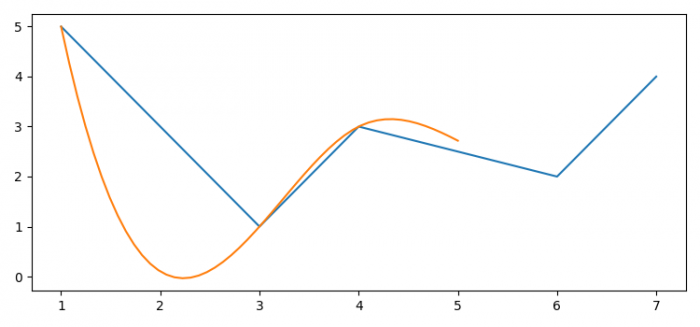# How to plot a smooth line with matplotlib?

To plot a smooth line with matplotlib, we can take the following steps −

## Steps

• Set the figure size and adjust the padding between and around the subplots.

• Create a list of data points, x and y.

• Plot the x and y data points.

• Create x_new and bspline data points for smooth line.

• Get y_new data points. Compute the (coefficients of) interpolating B-spline.

• Plot x_new and y_new data points using plot() method.

• To display the figure, use show() method.

## Example

import numpy as np
from matplotlib import pyplot as plt
from scipy import interpolate

# Set the figure size
plt.rcParams["figure.figsize"] = [7.50, 3.50]
plt.rcParams["figure.autolayout"] = True

# x and y data points
x = np.array([1, 3, 4, 6, 7])
y = np.array([5, 1, 3, 2, 4])

# Plot the data points
plt.plot(x, y)

# x_new, bspline, y_new
x_new = np.linspace(1, 5, 50)
bspline = interpolate.make_interp_spline(x, y)
y_new = bspline(x_new)

# Plot the new data points
plt.plot(x_new, y_new)

plt.show()

## Output

It will produce the following output −